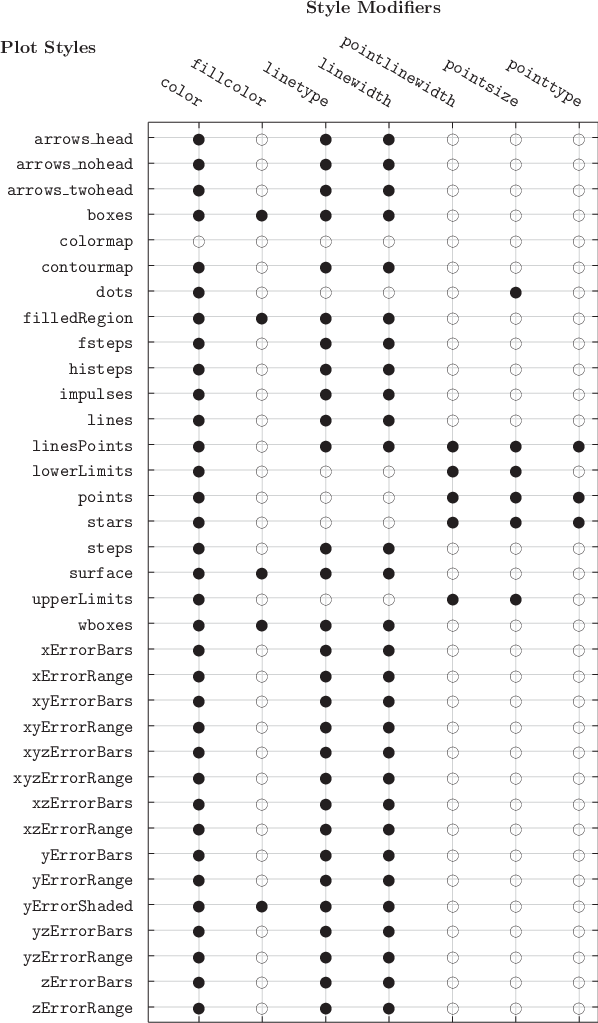# 8.1 The with modifier

In Chapter 3 an overview of the syntax of the plot command was provided, including the every, index, select and using modifiers, which can be used to control which data should be plotted. The with modifier controls how data should be plotted. For example, the statement

```plot "data.dat" index 1 using 4:5 with lines
```

specifies that data should be plotted with lines connecting each data point to its neighbors. We term the keyword lines a plot style. The with modifier can also be followed by a variety of settings which fine-tune aspects of how data are displayed. For example, the statement

```plot "data.dat" with lines linewidth 2.0
```

would connect data points with a line of twice the default width.

The next section will provide a complete list of all of Pyxplot’s plot styles – i.e. the words which may be used in place of lines. First we list all of the modifiers such as line­width which may be used to alter the exact appearance of these plot styles. These are as follows:

• color – used to select the color in which the dataset is to be plotted. It should be followed either by a number, to select a color from the present palette (see Section 8.1.1); by a recognised color name, a complete list of which can be found in Section 19.4; or by a color object, such as may be created by the functions gray(g), rgb(r,g,b), cmyk(c,m,y,k) or hsb(h,s,b). This modifier may also be spelt colour.

• fillcolor – used to select the color in which the dataset is filled. The color may be specified using any of the styles listed for color. May also be spelt fillcolour.

• linetype – used to numerically select the type of line – for example, solid, dotted, dashed, etc. – which should be used by line-based plot styles. A complete list of Pyxplot’s numbered line types can be found in Chapter 18. May be abbreviated lt.

• linewidth – used to select the width of line which should be used by line-based plot styles, where unity represents the default width. May be abbreviated lw.

• pointlinewidth – used to select the width of line which should be used to stroke points in point-based plot styles, where unity represents the default width. May be abbreviated plw.

• pointsize – used to select the size of drawn points, where unity represents the default size. May be abbreviated ps.

• pointtype – used to numerically select the type of point – for example, crosses, circles, etc. – used by point-based plot styles. A complete list of Pyxplot’s numbered point types can be found in Chapter 18. May be abbreviated pt.

Any number of these modifiers may be placed sequentially after the keyword with, as in the following examples:

```plot 'datafile' using 1:2 with points pointsize 2
plot 'datafile' using 1:2 with lines color red linewidth 2
plot 'datafile' using 1:2 with lp col 1 lw 2 ps 3
```

Where modifiers take numerical values, expressions of the form \$2+1, similar to those supplied to the using modifier, may be used to read numbers from the supplied data set. In this case, each datapoint will be displayed in a different style or in a different color (in the example given, depending on the values in the second column of the supplied data).

The following example would plot a data file with points, drawing the position of each point from the first two columns of the supplied data file and the size of each point from the third column:

```plot 'datafile' using 1:2 with points pointsize \$3
```

Not all of these modifiers are applicable to all of Pyxplot’s plot styles. For example, the line­width modifier has no effect on plot styles which do not draw lines between datapoints. Where modifiers are applied to plot styles for which they have no defined effect, the modifier has no effect, but no error results. Table 8.1 lists which modifiers act on which plot styles.Table 8.1: A list of the plot styles affected by each style modifiers.